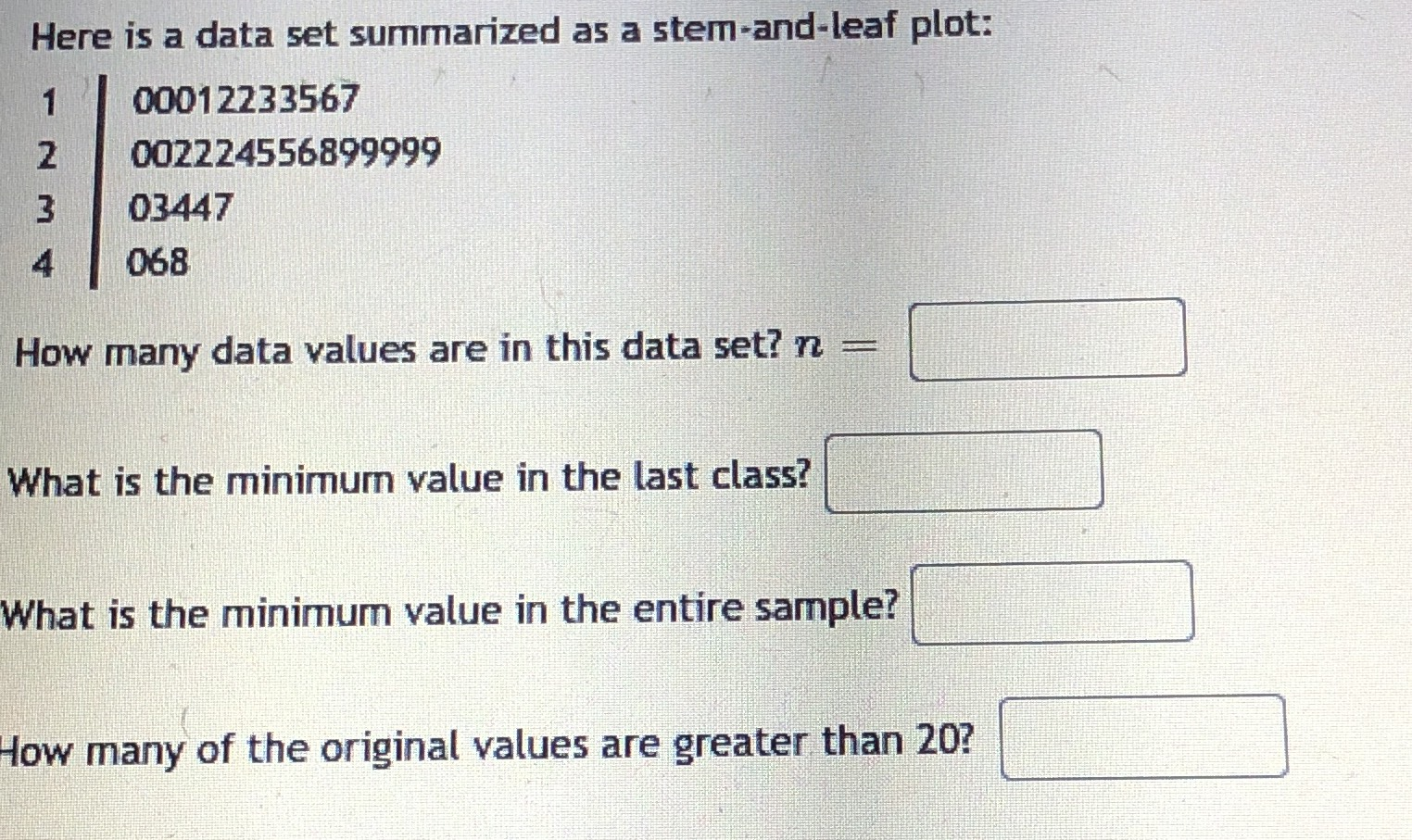### Still have math questions?

Arithmetic
QuestionHere is a data set summarized as a stem-and-leaf plot:

$$00012233567$$

$$002224556899999$$

$$03447$$

$$068$$

How many data values are in this data set? $$n =$$

What is the minimum value in the last class?

What is the minimum value in the entire sample?

How many of the original values are greater than $$20$$ ?